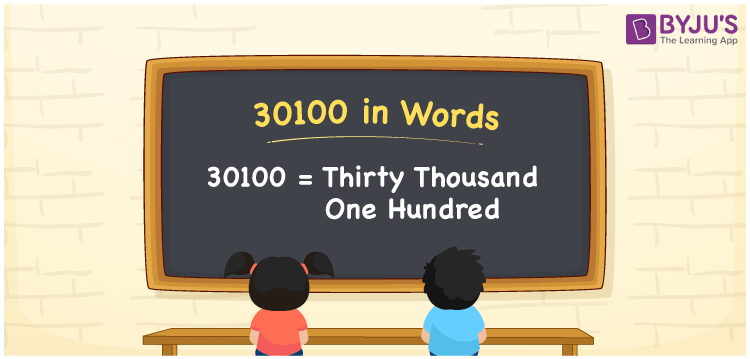# 30100 in Words

30100 in words is written as Thirty thousand one hundred. In both the International System of Numerals and the Indian System of Numerals, 30100 is written as Thirty thousand one hundred. The number 30100 is a Cardinal Number as it could represent some quantity. For example, “that laptop costs 30100 rupees”.

 30100 in Words Thirty thousand one hundred Thirty thousand one hundred in Number 30100

## 30100 in English Words

30100 in English words is read as “Thirty thousand one hundred”.## How to Write 30100 in Words?

To write 30100 in words, we shall use the place value chart. In the place value chart, put 3 in the ten thousands, 1 in the hundreds, 0 in the thousands, tens and ones. Let us make a place value chart to write the number 30100 in words.

 Ten Thousands Thousands Hundreds Tens Ones 3 0 1 0 0

Thus, we can write the expanded form as

3 × Ten Thousand + 0 × Thousand + 1 × Hundred + 0 × Ten + 0 × One

= 3 × 10000 + 0 × 1000 + 1 × 100 + 0 × 10 + 0 × 1

= 30000 + 0 + 100 + 0 + 0

= 30100

= Thirty thousand one hundred.

30100 is a natural number, the successor of 30099 and the predecessor of 30101.

30100 in words – Thirty thousand one hundred

• Is 30100 an odd number? – No
• Is 30100 an even number? – Yes
• Is 30100 a perfect square number? – No
• Is 30100 a perfect cube number? – No
• Is 30100 a prime number? – No
• Is 30100 a composite number? – Yes

## Frequently Asked Questions on 30100 in Words

Q1

### How to write 30100 in words?

30100 in words is written as Thirty thousand one hundred.
Q2

### How to write 30100 in the International and Indian System of Numerals?

In both, the system of numerals, 30100 in words, is written as Thirty thousand one hundred.
Q3

### How to write 30100 in a place value chart?

In the place value chart, 3 in the ten thousands, 1 in the hundreds, 0 in the thousands, tens and ones.